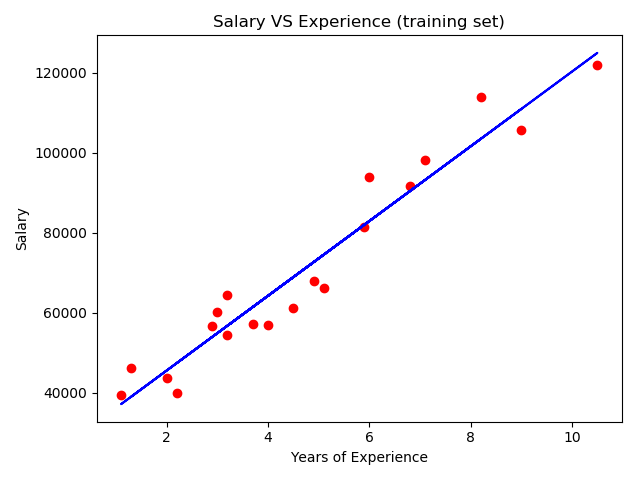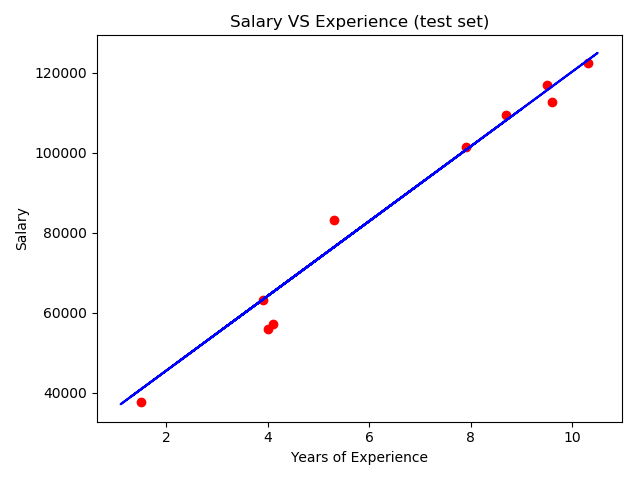# 机器学习A-Z～简单线性回归

YearsExperience,Salary
1.1,39343.00
1.3,46205.00
1.5,37731.00
2.0,43525.00
2.2,39891.00
2.9,56642.00
3.0,60150.00
3.2,54445.00
3.2,64445.00
3.7,57189.00
3.9,63218.00


import pandas as pd
from sklearn.model_selection import train_test_split
from sklearn.linear_model import LinearRegression
import matplotlib.pyplot as plt

data_path = '../simple_regression/data/Salary_Data.csv'

X = dataset.iloc[:, :-1].values
y = dataset.iloc[:, 1].values

X_train, X_test, y_train, y_test = train_test_split(X, y, test_size=1/3, random_state=0)


# Fitting Simple Linear Regression to the Training Set
regressor = LinearRegression()
regressor.fit(X_train, y_train)


# Predicting the Test set results
y_pred = regressor.predict(X_test)


#Visualising the Training set results
plt.scatter(X_train, y_train, c='red')
plt.plot(X_train, regressor.predict(X_train), c='blue')
plt.title('Salary VS Experience (training set)')
plt.xlabel('Years of Experience')
plt.ylabel('Salary')
plt.show()

#Visualising the test set results
plt.scatter(X_test, y_test, c='red')
plt.plot(X_train, regressor.predict(X_train), c='blue')
plt.title('Salary VS Experience (test set)')
plt.xlabel('Years of Experience')
plt.ylabel('Salary')
plt.show()


plt.plot(X_train, regressor.predict(X_train), c='blue')